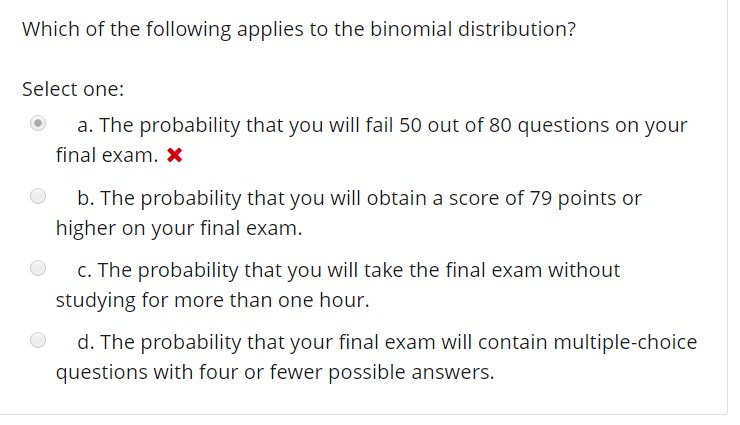# Which of the following applies to the binomial distribution? Select one: a. The probability that you will fail 50 out of 80 questions on your b. The probability that you will obtain a score of 79 points or C. The probability that you will take the final exam without d. The probability that your final exam will contain multiple-choice final exam. x higher on your final exam. studying for more than one hour. questions with four or fewer possible answers.

Questionhelp_outlineImage TranscriptioncloseWhich of the following applies to the binomial distribution? Select one: a. The probability that you will fail 50 out of 80 questions on your b. The probability that you will obtain a score of 79 points or C. The probability that you will take the final exam without d. The probability that your final exam will contain multiple-choice final exam. x higher on your final exam. studying for more than one hour. questions with four or fewer possible answers. fullscreen